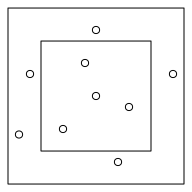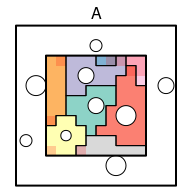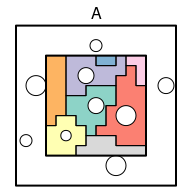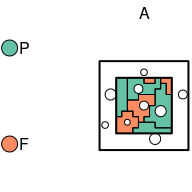# APAtree-vignette

## Introduction

The APAtree-package calculates maps of the the ‘area potentially available’ to trees (APA-maps) out of tree inventory data from one or more research plots. The APA is a measure for the growing space of trees that sums up to the stand area (Gspaltl et al. 2012, Römisch 1995). This is done by computing a rasterized version of ‘weighted voronoi diagrams’ using an approximation of the trees competitive ability (e.g., crown radius, leaf area) as weight. Based on APA-maps, several APA-properties that describe tree proportions and diversity of complete forest stands or of neighborhoods around individual trees are implemented in this package (Gspaltl. et al. 2012, Glatthorn 2021). This Vignette is a step by step user guide about how to use the most important functions of the APAtree-package. For methodological details please consult the original references.

## Creating some example data

To exemplarily demonstrate the functionality of APAtree, first some data that has the typical structure of tree inventory data is required.

The APAtree-package calculates maps of the the ‘area potentially available’ to trees (APA-maps) out of tree inventory data from one or more research plots. The APA is a measure for the growing space of trees that sums up to the stand area (Gspaltl et al. 2012). In this package the approach to derive APA-maps from Gspaltl et al. (2012) that is based on a rasterized version of weighted Voronoi diagrams is implemented. In this approach, the complete stand area is rasterized into a regular grid and each grid cell is assigned to the closest tree, weighted by a tree trait that approximates the trees competitive ability (e.g., the crown radius). Based on APA-maps, several APA-properties that describe tree proportions and diversity of complete forest stands or of neighborhoods around individual trees are implemented in this package (Gspaltl. et al. 2012, Glatthorn 2021). This Vignette is a step by step user guide about how to use the most important functions of the APAtree-package, for methodological details please consult the original references.

## Creating some example data

To exemplarily demonstrate the functionality of , first some data that has the typical structure of tree inventory data is required.

library(APAtree)
#> Linking to GEOS 3.6.2, GDAL 2.2.3, PROJ 4.9.3

# invent some tree data
example_tree_dat <-
data.frame(id_tree = as.character(1:9),
id_plot = "A",
weight = c(4, 2.6, 4, 5, 3, 5, 3, 4, 5),
species = c("F", "F", "P", "P", "F", "P", "F", "F", "P"),
x = c(5, 2, 4, 8, 5, -1, -2, 12, 7),
y = c(5, 2, 8, 4, 11, 7, 1.5, 7, -1))

This example dataset contains a minimum amount of variable to create APA-maps:

• id_tree: A unique tree id (character), no two trees are allowed to have the same id.
• id_plot: A plot id (character). A second dataset about the layout of the research plots necessary to calculate APA-maps. The datasets will be linked via the plot id column.
• weight: A numeric positive variable that is used as weight to account for trees with different competitive abilities (mainly due to tree size). In real datasets, a trait that approximates the competitive ability such as the crown radius, diameter or leaf area should be chosen.
• species (optional): A character variable to group certain trees (does not necessarily have to be species, could be tree size classes as well).
• x and y: numeric variables to specify tree coordinates. The coordinates can be in a local coordinate system (e.g., relative to the plot center or plot corner) or they can be in a geographic coordinate reference system. The reference system has to be metric.

The tree data is spatial vector data. The sf-package is used by APAtree to work with spatial data. First, the tree data needs to be converted to sf-objects. If tree and plot data is available as geodata, it can directly be imported using the sf::st_read()-function as well.

example_tree_dat <- st_as_sf(example_tree_dat, coords = c("x", "y"))

Additionally, the plot area needs to be specified as POLYGON-data, again as sf-object. If available, a buffer area surrounding the actual core plot where additional tree data was recorded to avoid edge-effects may be specified (plus-sampling).

# A test plot area surrounding the threes
plot_poly <-
st_polygon(x = list(matrix(c(0, 0, 0, 10, 10, 10, 10, 0, 0, 0),
ncol = 2, byrow = TRUE))) %>%
st_sfc()

buffer_poly <-
st_polygon(x = list(matrix(c(-3, -3, -3, 13, 13, 13, 13, -3, -3, -3),
ncol = 2, byrow = TRUE))) %>%
st_sfc()

example_plot_dat <-
st_sf(id_plot = "A",
buffer_geometry = buffer_poly,
border_geometry = plot_poly)

The plot dataset only has three relevant columns:

• id_plot The unique plot id that is used to relate tree and plot data;
• buffer_geometry The total area where trees were recorded as sfc_POLYGON-column;
• border_geometry The actual core area of interest as sfc_POLYGON-column.
par(mai = rep(0.01, 4))
plot(example_plot_dat$buffer_geometry) plot(example_plot_dat$border_geometry, add = TRUE)
plot(example_tree_dat$geometry, add = TRUE)## Create and plot an apa_list The main work is done by the apa_list-function that returns an object of class apa_list. example_apa_list <- apa_list(tree_dat = example_tree_dat, plot_dat = example_plot_dat, plot_id_column = "id_plot", tree_id_column = "id_tree", core_column = "border_geometry", buffer_column = "buffer_geometry", weight_column = "weight", apa_polygon = TRUE) #> Generating APA-maps: #> A #> #> Starting polygonization: #> tree_dat - polygonizing APA-patches: #> A print(example_apa_list) #> List with APA-maps of 1 plot and 9 trees. #> plot id column: id_plot ("A") #> bbox: xmin: 0 xmax: 10 #> ymin: 0 ymax: 10 #> CRS: NA #> resolution: 1 The actual APA-maps are stored as a list of RasterStack-objects from the raster-package (?raster::stack) in the apa_list. example_apa_list$plot_dat$apa_map #>$A
#> class      : RasterStack
#> dimensions : 10, 10, 100, 2  (nrow, ncol, ncell, nlayers)
#> resolution : 1, 1  (x, y)
#> extent     : 0, 10, 0, 10  (xmin, xmax, ymin, ymax)
#> crs        : NA
#> names      : id_tree, critical
#> min values :       1,        0
#> max values :       8,        1

There are at least two layers in each APA-map. The first one is the layer containing the APA-patches of individual trees, the second one is a binary layer called critical. The critical-layer contains information about potential edge-effects on the assignment of raster cells to trees. If a cell would be assigned to a large tree (as large as the largest one in the dataset) that may be located directly at the outer buffer-border, a raster cell is considered critical.

To display the final apa_list graphically in different ways, use the plot-function (see ?plot.apa_list for more information on how to configure the plot).

# Default settings
plot(example_apa_list)# Do not color the critical area
plot(example_apa_list, critical  = NA)# Use custom colors
plot(example_apa_list, critical  = NA,
color_map =
data.frame(species = c("P", "F"),
color = sf::sf.colors(2, categorical = TRUE)))## Calculation of APA-properties

### APA-size

The most straight forward property from an APA-map is the size of APA-patches, which can be calculated using the apa_size() function.

example_apa_list_2 <- apa_size(example_apa_list)
#>
#> Aggregating APA-sizes:
#> A

# The absolute size of the APA-patches within the core plot of all trees (NA means that the tree doesn't have an APA within the core plot).
example_apa_list_2$tree_dat$apa_size
#>  16 13 17 25  2 13 NA  4 10

# The relative size of the APA-patches within the core plot of all trees (NA means that the tree doesn't have an APA within the core plot).
example_apa_list_2$tree_dat$apa_size_prop
#>  0.16 0.13 0.17 0.25 0.02 0.13   NA 0.04 0.10

# Information about the absolute size of the critical area of each tree
example_apa_list_2$tree_dat$critical_area
#>   0  1  1  1  1  2 NA  3  1

# Information about the proportion of the total apa size of a tree that is critical
example_apa_list_2$tree_dat$critical
#>  0.00000000 0.07692308 0.05882353 0.04000000 0.50000000 0.15384615         NA
#>  0.75000000 0.10000000

# The critical area may be ignored when calculating the APA-size:
example_apa_list_3 <- apa_size(example_apa_list, edge_correction = "critical")
#>
#> Aggregating APA-sizes:
#> A

# In this case, the APA-size gets smaller:
example_apa_list_2$tree_dat$apa_size - example_apa_list_3$tree_dat$apa_size
#>   0  1  1  1  1  2 NA  3  1

# The apa_size_total column always gives the overall APA-size irrespective of the used edge-correction method:
example_apa_list_3$tree_dat$apa_size_total
#>  16 13 17 25  2 13 NA  4 10

# The relative apa size is always calculated relative to the APA-size with edge-correction:
all.equal(example_apa_list_3$tree_dat$apa_size /
sum(example_apa_list_3$tree_dat$apa_size, na.rm = TRUE),
example_apa_list_3$tree_dat$apa_size_prop)
#>  TRUE

### Neighborhood diversity NDiv

The species neighborhood diversity SpeciesNDiv is calculated with the apa_ndiv() function, using the species-column as trait to calculate dissimilarity. At tree-level, SpeciesNDiv is the proportion of mixed borders that a tree shares with its neighbors. Other numeric and/or categorical columns may be supplied to calculate neighborhood diversity regarding other sets of traits (see ?apa_ndiv for more details).

example_apa_list_4 <- apa_ndiv(example_apa_list, dis_trait_column = "species")
#>
#> Aggregating APA-sizes:
#> A
#>
#> Aggregating species neighborhood diversity:A

# SpeciesNDiv of the trees (proportion of mixed borders surrounding each tree):
example_apa_list_4$tree_dat$species_ndiv
#>  0.8000000 0.6000000 0.6111111 0.5500000 1.0000000 0.5555556        NA
#>  1.0000000 0.4000000

# SpeciesNDiv at stand-level:
example_apa_list_4$plot_dat$species_ndiv
#>  0.6196111
# Which is the weighted average of all individual SpeciesNDiv values:
weighted.mean(example_apa_list_4$tree_dat$species_ndiv,
example_apa_list_4$tree_dat$apa_size,
na.rm = TRUE)
#>  0.6196111
# As the APA-size is required for upscaling, it is always added before calculating NDiv.

if pdiv = TRUE, the proportion-based diversity (that ignores the spatial configuration of the stand) is provided as well. In case of the species diversity at stand-level, this would be Simpson diversity, for example.

# pdiv
(pdiv = example_apa_list_4$plot_dat$species_pdiv)
#>  0.455

# Simpson diversity using APA-sizes
species_apa_size <-
tapply(example_apa_list_4$tree_dat$apa_size,
example_apa_list_4$tree_dat$species,
sum, na.rm = TRUE)
(simpson <- 1 - sum((species_apa_size / 100)^2))
#>  0.455
all.equal(pdiv, simpson)
#>  TRUE

### Aggregating APA-properties at species-level

Aggregation of APA-properties is done with the apa_add_agg_class() function. In this example, values are aggregated at species-level. Other tree related categories (e.g., height_classes) that are stored in the tree data set may be used as well.

# The aggregation classes are specifies as columns of the tree data
example_apa_list_5 <-
apa_add_agg_class(example_apa_list, agg_class_column = "species")
#>
#> Adding aggregation classes:
#> species
#> tree_dat - polygonizing APA-patches:
#> A
#> species - polygonizing APA-patches:  A

All APA-properties are calculated for the aggregation classes as well

example_apa_list_6 <-
apa_ndiv(example_apa_list_5, dis_trait_column = "species")
#>
#> Aggregating APA-sizes:
#> A
#>
#> Aggregating species neighborhood diversity:A
example_apa_list_6\$species[, c("species", "species_ndiv")]
#> Simple feature collection with 2 features and 2 fields
#> Geometry type: MULTIPOLYGON
#> Dimension:     XY
#> Bounding box:  xmin: 0 ymin: 0 xmax: 10 ymax: 10
#> CRS:           NA
#>   species species_ndiv                    apa_polygon
#> 1       F    0.7600000 MULTIPOLYGON (((5 10, 7 10,...
#> 3       P    0.5440171 MULTIPOLYGON (((0 10, 5 10,...